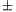Mathematical and Physical Journal
for High Schools
Issued by the MATFUND Foundation
 Already signed up? New to KöMaL?

#Problem A. 498. (January 2010)

A. 498. Let p(x) be a polynomial with integer coefficients, and let w be a complex number of unit absolute value. Prove that if the number c=p(w) is purely real, then there is a polynomial q(x) with integer coefficients for which.

(5 pont)

Deadline expired on February 10, 2010.

Solution. If w=1 then p(w) is an integer, and the statement is trivial. So we can assume that w is not real.

Lemma. For every k nonnegative integer there exists a polynomial uk(x) such that

sin (k+1)t=uk(2cos t).sin t.

Proof. We apply induction on k.

In the cases k=0 and k=1 the polynomials u0(x)=1 and u1(x)=x satisfy the conditions, since sin t=1.sin t and sin 2t=2cos t.sin t.

If we already have uk-1(x) and uk-2(x) then define

uk(x)=uk-1(x).x-uk-2(x).

This polynomial has integer coefficients too, and

sin (k+1)t=2sin ktcos t-sin (k-1)t=2.uk-1(2cos t)sin t.cos t-uk-2(2cos t)sin t=

=(uk-1(2cos t).2cos t-uk-2(2cos t))sin t=uk(2cos t)sin t.

The Lemma is complete

Ifthen let.

If w=cos t+isin t, where by our assumption, thenRemark. The problem is closely related to the 6th problem of CIIM 1. In the solution above we used the ideas of Pablo Soberon Bravo (Mexiko).

### Statistics:

 7 students sent a solution. 5 points: Backhausz Tibor, Bodor Bertalan, Éles András, Nagy 235 János, Nagy 648 Donát, Strenner Péter, Szabó 928 Attila.

Problems in Mathematics of KöMaL, January 2010